## 2.1 Significant Figures

### Learning Objectives

By the end of this section, you will be able to:

• Apply the concept of significant figures to limit a measurement to the proper number of digits.
• Recognize the number of significant figures in a given quantity.
• Limit mathematical results to the proper number of significant figures.

## Uncertainty of Measurement

If you use a calculator to evaluate the expression 337 ÷ 217, you will get the following:

337 ÷ 217 = 1.55299539171…

and so on for many more digits.  Although this answer is correct, it is somewhat presumptuous. You start with two values that each have three digits, and the answer has twelve digits?  That does not make much sense from a strict numerical point of view.

Consider using a graduated cylinder to measure the volume of a liquid, as shown in Figure 2.1. The numbers of measured quantities, unlike defined or directly counted quantities, are not exact. To measure the volume of liquid in a graduated cylinder, you should make a reading at the bottom of the meniscus, the lowest point on the curved surface of the liquid.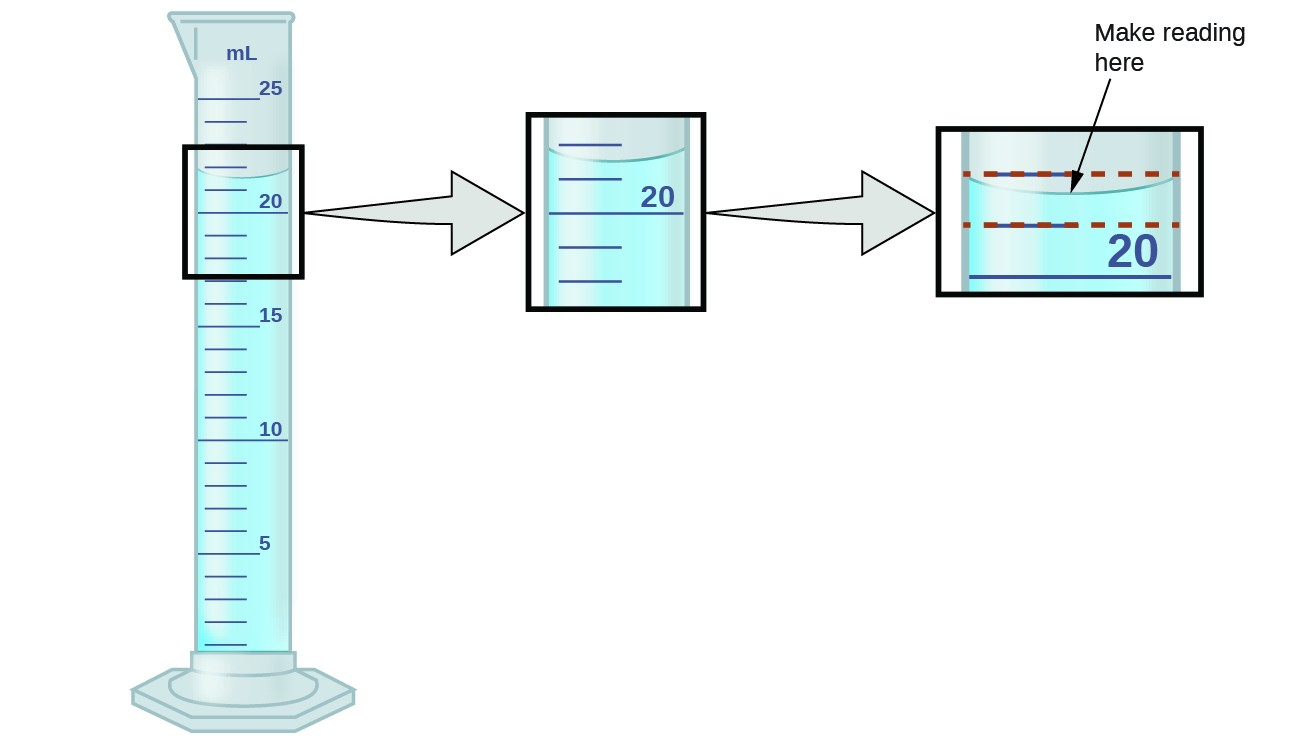Figure 2.1. To measure the volume of liquid in this graduated cylinder, you must mentally subdivide the distance between the 21 and 22 mL marks into tenths of a milliliter, and then make a reading (estimate) at the bottom of the meniscus.

Refer to the illustration in Figure 2.1. The bottom of the meniscus in this case clearly lies between the 21 and 22 markings, meaning the liquid volume is certainly greater than 21 mL but less than 22 mL. The meniscus appears to be a bit closer to the 22-mL mark than to the 21-mL mark, and so a reasonable estimate of the liquid’s volume would be 21.6 mL. In the number 21.6, then, the digits 2 and 1 are certain, but the 6 is an estimate. Some people might estimate the meniscus position to be equally distant from each of the markings and estimate the tenth-place digit as 5, while others may think it to be even closer to the 22-mL mark and estimate this digit to be 7. Note that it would be pointless to attempt to estimate a digit for the hundredths place, given that the tenths-place digit is uncertain. In general, numerical scales such as the one on this graduated cylinder will permit measurements to one-tenth of the smallest scale division. The scale in this case has 1-mL divisions, and so volumes may be measured to the nearest 0.1 mL.

This concept holds true for all measurements, even if you do not actively make an estimate. If you place a quarter on a standard electronic balance, you may obtain a reading of 6.72 g. The digits 6 and 7 are certain, and the 2 indicates that the mass of the quarter is likely between 6.71 and 6.73 grams. The quarter weighs about 6.72 grams, with a nominal uncertainty in the measurement of ± 0.01 gram. If we weigh the quarter on a more sensitive balance, we may find that its mass is 6.723 g. This means its mass lies between 6.722 and 6.724 grams, an uncertainty of 0.001 gram. Every measurement has some uncertainty, which depends on the device used (and the user’s ability). All of the digits in a measurement, including the uncertain last digit, are called significant figures or significant digits. Note that zero may be a measured value; for example, if you stand on a scale that shows weight to the nearest pound and it shows “120,” then the 1 (hundreds), 2 (tens) and 0 (ones) are all significant (measured) values.

### Example 1: uncertainty of Measurements

1. Record the following measurement to the proper number of significant figures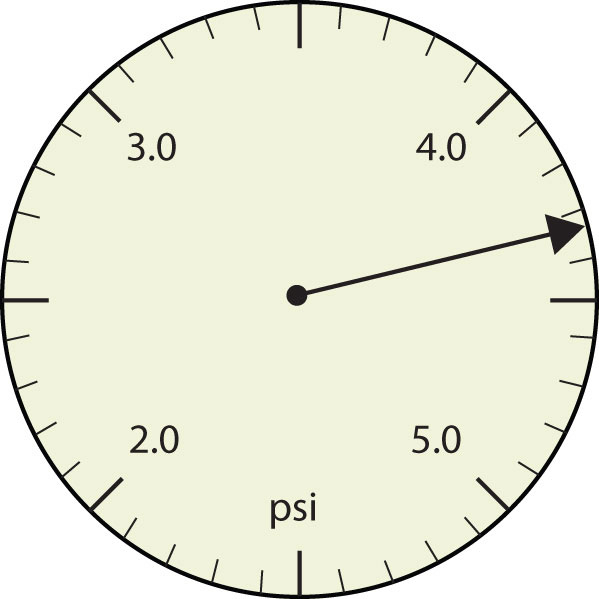2. Record the following measurement to the proper number of significant figures

What would be the reported width of this rectangle?

## How Many Significant Figures are in a Given Measurement?

In many cases, you will be given a measurement. How do we know what digits are significant?  For example, the reported population of the United States is 306,000,000.  Does that mean that it is exactly three hundred six million or is some estimation occurring? Nonzero digits are always significant figures.  However, a zero may or not be significant.

The following conventions dictate which numbers in a reported measurement are significant and which are not significant:

 Significant Figures in Numbers Examples (significant figures in bold) Any nonzero digit is significant. 13.4 (three significant figures) Any zeros between nonzero digits (i.e., embedded zeros) are significant 404.1 (four significant figures) Leading zeros (zeros to the left of the first nonzero digit) are not significant. 0.000021 (two significant figures) Trailing zeros (zeros at the end of a number) without a decimal point are not significant. 4000 (1 significant figure) Trailing zeros with a decimal point are significant. 4000.0 (5 significant figures)

So, by these rules, the population figure of the United States has only three significant figures: the 3, the 6, and the zero between them.  The remaining six zeros simply put the 306 in the millions position.

### Example 2: Significant figures in measurements

Give the number of significant figures in each measurement.

1. 36.7 m
2. 0.006606 s
3. 2,002 kg
4. 306,490,000 people

Give the number of significant figures in each measurement.

1. 0.000601 m
2. 65.080 kg

## Significant Figures in Calculations

How are significant figures handled in calculations?  It depends on what type of calculation is being performed.  If the calculation is an addition or a subtraction, the rule is as follows: limit the reported answer to the rightmost column that all numbers have significant figures in common.  For example, if you were to add 1.2 and 4.71, we note that the first number stops its significant figures in the tenths column, while the second number stops its significant figures in the hundredths column.  We therefore limit our answer to the tenths column.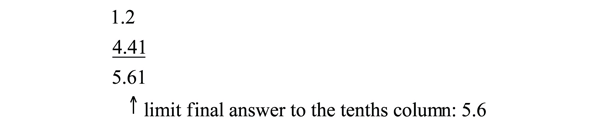We drop the last digit—the 1—because it is not significant to the final answer.

The dropping of positions in sums and differences brings up the topic of rounding.  Although there are several conventions, in this text we will adopt the following rule: the final answer should be rounded up if the first dropped digit is 5 or greater and rounded down if the first dropped digit is less than 5.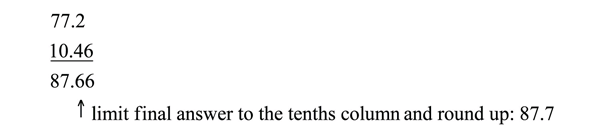### Example 3: Rounding

Round the following to the indicated number of significant figures:

1. 31.67 (to two significant figures)
2. 8.1649 (to three significant figures)
3. 0.90275 (to four significant figures)

Round the following to the indicated number of significant figures:

1. 0.424 (to two significant figures)
2. 0.0038661 (to three significant figures)
3. 421.25 (to four significant figures)
4. 28,683.5 (to five significant figures)

### Example 4: Addition and Subtraction with Significant Figures

Rule: When we add or subtract numbers, we should round the result to the same number of decimal places as the number with the least number of decimal places (i.e., the least precise value in terms of addition and subtraction).

1. Add 1.0023 g and 4.383 g.
2. Subtract 421.23 g from 486 g.

1. Add 2.334 mL and 0.31 mL.
2. Subtract 55.8752 m from 56.533 m.

If the operations being performed are multiplication or division, the rule is as follows: limit the answer to the number of significant figures that the data value with the least number of significant figures has.  So if we are dividing 23 by 448, which have two and three significant figures each, we should limit the final reported answer to two significant figures (the lesser of two and three significant figures):

23 ÷ 448 = 0.051339286… = 0.051

The same rounding rules apply in multiplication and division as they do in addition and subtraction.

### Example 5: Multiplication and Division with Significant Figures

Rule: When we multiply or divide numbers, we should round the result to the same number of digits as the number with the least number of significant figures (the least precise value in terms of multiplication and division).

1. Multiply 0.6238  by 6.6
2. Divide 421.23 by 486

1. Multiply 2.334 and 0.320
2. Divide 55.8752 by 56.53

As you have probably realized by now, the biggest issue in determining the number of significant figures in a value is the zero.  Is the zero significant or not?  One way to unambiguously determine whether a zero is significant or not is to write a number in scientific notation. Scientific notation will include zeros in the coefficient of the number only if they are significant.  Thus, the number 8.666 × 106 has four significant figures.  However, the number 8.6660 × 106 has five significant figures.  That last zero is significant; if it were not, it would not be written in the coefficient.  So when in doubt about expressing the number of significant figures in a quantity, use scientific notation and include the number of zeros that are truly significant.

Video source: Significant figures by keyj (https://viuvideos.viu.ca/media/Significant+Figures/0_t8xwe4s9)

### Key Takeaways

• Significant figures in a quantity indicate the number of known values plus one place that is estimated.
• There are rules for which numbers in a quantity are significant and which are not significant.
• In calculations involving addition and subtraction, limit significant figures based on the rightmost place that all values have in common.
• In calculations involving multiplication and division, limit significant figures to the least number of significant figures in all the data values.

### Exercises

1. Express each measurement to the correct number of significant figures.

a)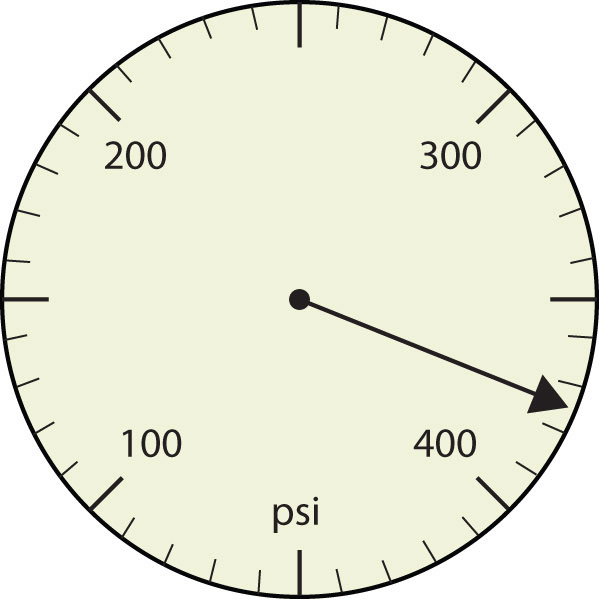b)

2.  Express each measurement to the correct number of significant figures.

a)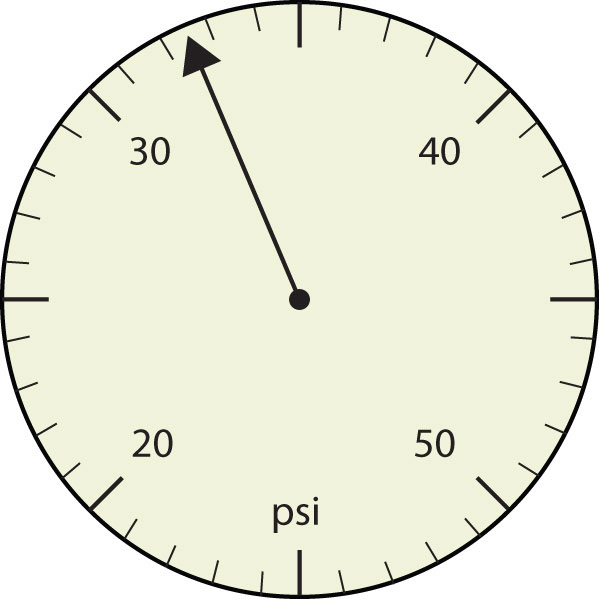b)

3.  How many significant figures do these numbers have?

a)  23

b)  23.0

c)  0.00023

d)  0.0002302

4.  How many significant figures do these numbers have?

a)  5.44 × 108

b)  1.008 × 10−5

c)  43.00

d)  0.00000013810

5.  How many significant figures do these numbers have?

a)  765,890

b)  765,890.0

c)  1.2000 × 105

d)  0.0005060

6)  How many significant figures do these numbers have?

a)  0.009

b)  0.0000009

c)  65,444

d)  65,040

7.  Compute and express each answer with the proper number of significant figures, rounding as necessary.

a)  56.0 + 3.44 = ?

b)  0.00665 + 1.004 = ?

c)  45.99 − 32.8 = ?

d)  45.99 − 32.8 + 75.02 = ?

8.  Compute and express each answer with the proper number of significant figures, rounding as necessary.

a)  1.005 + 17.88 = ?

b)  56,700 − 324 = ?

c)  405,007 − 123.3 = ?

d)  55.5 + 66.66 − 77.777 = ?

9.  Compute and express each answer with the proper number of significant figures, rounding as necessary.

a)  56.7 × 66.99 = ?

b)  1.000 ÷ 77 = ?

c)  1.000 ÷ 77.0 = ?

d)  6.022 × 1.89 = ?

10.  Compute and express each answer with the proper number of significant figures, rounding as necessary.

a)  0.000440 × 17.22 = ?

b)  203,000 ÷ 0.044 = ?

c)  67 × 85.0 × 0.0028 = ?

d)  999,999 ÷ 3,310 = ?

11.  Write the number 87,449 in scientific notation with four significant figures.

12.  Write the number 0.000066600 in scientific notation with five significant figures.

13.  Write the number 306,000,000 in scientific notation to the proper number of significant figures.

14.  Write the number 0.0000558 in scientific notation with two significant figures.

## Glossary

exact number: number derived by counting or by definition

precision: how closely a measurement matches the same measurement when repeated

rounding: procedure used to ensure that calculated results properly reflect the uncertainty in the measurements used in the calculation

significant figures: (also, significant digits) all of the measured digits in a determination, including the uncertain last digit

uncertainty: estimate of amount by which measurement differs from true value